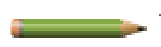# Evaluating alternative notes A borrower has two alternatives for a loan: (1) issue a $360,000, 60-day, 5% note or (2) issue a$360,000, 60-day note that the creditor discounts at 5%. a. Calculate the amount of the interest expense for each option. b. Determine the proceeds received by the borrower in each situation. c. Which alternative is more favorable to the borrower? Explain.### Financial Accounting

15th Edition
Carl Warren + 2 others
Publisher: Cengage Learning
ISBN: 9781337272124

#### Solutions

Chapter
Section### Financial Accounting

15th Edition
Carl Warren + 2 others
Publisher: Cengage Learning
ISBN: 9781337272124
Chapter 11, Problem 4E
Textbook Problem
12 views

## Evaluating alternative notesA borrower has two alternatives for a loan: (1) issue a $360,000, 60-day, 5% note or (2) issue a$360,000, 60-day note that the creditor discounts at 5%. a. Calculate the amount of the interest expense for each option. b. Determine the proceeds received by the borrower in each situation. c.Which alternative is more favorable to the borrower? Explain.

a.

To determine

Calculate the amount of interest expense for each option.

### Explanation of Solution

Notes Payable: Notes Payable is a written promise to pay a certain amount on a future date, with certain percentage of interest. Companies use to issue notes payable to meet short-term financing needs.

Calculate the amount of interest expense for Alternative 1.

Interest Expense = Note Payable×Interest Rate×Interettimeperiods                          = $360,000×5%×60360 =$3,000

Calculate the amount of interest expense for Alternative 2

b.

To determine

Calculate the proceeds amount received by borrower in each Alternative.

c.

To determine

Choose the more favorable Alternative to the borrower.

### Still sussing out bartleby?

Check out a sample textbook solution.

See a sample solution

#### The Solution to Your Study Problems

Bartleby provides explanations to thousands of textbook problems written by our experts, many with advanced degrees!

Get Started

Find more solutions based on key concepts
What are the arguments against increased social responsibility?

Foundations of Business (MindTap Course List)

Distinguish between exposure and risk.

Accounting Information Systems

Why are interest charges not deducted when a project's cash flows for use in a capital budgeting analysis are c...

Fundamentals of Financial Management, Concise Edition (with Thomson ONE - Business School Edition, 1 term (6 months) Printed Access Card) (MindTap Course List)

12. How is a preferred stock dividend calculated?

Cornerstones of Financial Accounting